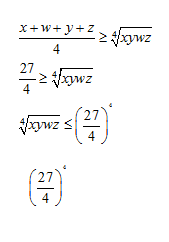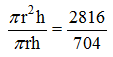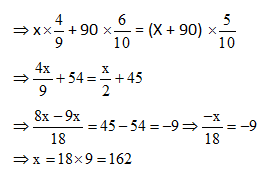# SSC CGL EXAMS 2018 | Quantitative Aptitude Practice Questions (Day-19)

Dear Aspirants, Here we have given the Important SSC Exam 2018 Practice Test Papers. Candidates those who are preparing for SSC 2018 can practice these questions to get more confidence to Crack SSC 2018 Examination.

[WpProQuiz 4813]

1) Which one of the following numbers will completely divide (623×824×525×226×127)?

a) 7

b) 10

c) 9

d) 11

2) Two fifth of a number is greater than one third by 11. Find the number.

a) 164

b) 165

c) 200

d) None of these

3) The sum of all odd numbers between 70 and 100 which are divisible by 9 is—

a) 160

b) 170

c) 252

d) None of these

4) Rahul bought a gift at 12.5% discount on the marked price and he sold it Raghu at a discount of 10% on the marked price. It Raghu paid Rs.900 for the gift they what percent of profit made by Rahul?

a) 2%

b) 20%

c) 28.5%

d) 2.85%

5) A 235 m long train running at a speed of 216km/h completely over takes a 115 m long train running in the same direction 10 seconds. The speed of the second train is:

a) 25 m/s

b) 20m/s

c) 10 m/s

d) 15 m/s

6) Find the minimum value of y = sin12x + cos12x.

a) 1/64

b) 1/32

c) 1/16

d) 1

7) Find the maximum value of xyzw, where x + y + z + w = 27.

a) 94

b) 49

c) (27/4)4

d) 9

8) The curved surface area of a cylindrical pillar is 704 cm2 and its volume is 2816 cm3. Find the ratio of the diameter of its base to its height.

a) 7 : 8

b) 8 : 7

c) 16 : 14

d) None of these

9) P, Q, R and S complete a work/ job is 10 days, 20 days, 25 days and 50 days respectively. P & Q start the work and working for one day only, and then Q left the Job, and in place of him R & S coin for the same job but after 2 days also let the job. Remaining work will be done by R & S only on the conditions of alternate day starting from R. In how many days work will complete?

a) 20 days

b) 20 ½ days

c) 16 days

d) 11. 83 days

10) How many liters of a solution containing milk and water in the ratio 4: 5 should be added to 90 liters of 60% milk solution in order to get 50% milk solution?

a) 160

b) 140

c) 162

d) 100

623×824×525×226×127

By product of unit digit of the above numbers

3×4×5×6×7 = 20 (last two digits)

∴ The product will be divisible by 10.

2x/5 = x/3 + 11

2x/3 = x + 33/3

6x = 5x + 165

x = 165

Odd numbers between 70 to 100 which are divisible by 9 is 81 & 99

Sum = 81 + 99 = 180

Let x be the marked price of the gift.

Raghu buys the gift for is 900, which is the price after @ 10% discount on marked price.

0.9x = 900

x = Rs. 1000

As Rahul bought the cycle at 12.5% discount, then amount paid by Rahul

= 1000  87.5% = 875 *1000/10 * 100 = Rs. 875

Profit made by Ragul = 900 – 875 = 25

Profit % = 25* 100/875 = 2.85%

The speed of the first train = 216 kmph = 54 * 5/ 18 = 60 mps

Let x (in m/s) is the speed of the second train.

(60 – x) * 10 = 235 + 115 = 350

600 – 10x = 350

10x = 250

x = 25 mps

For the value of y = sin12 x + cos12x (Here x = 45o)

So, (sin450)12 + (cos45o) 12

= (1/√2)12 + (1/√2)12 [sin 450 = 1/√2, cos 450= 1/√2]

= 1/64 + 1/64 = 2/ 64 = 1/32

By using AM  GM property;

[The AMGM inequality, states that the arithmetic mean of a list of non-negative real numbers is greater than or equal to the geometric mean of the same list]So, xyzw is Maximum for (27/4)4

Let r and h (in cm) be the radius of the base and the height of the pillar then,Diameter = 2r = 2 * 8 = 16 cm

πrh = 22/7 * 8 * h = 704

Height = 28cm

Radius : Diameter = 16 : 14 = 8 : 7A/c to Q. 1day work by P & Q = 10U+ 5U = 15U.

2 days’ work by P, R&S = 2 (10 + 4 + 2) units

Total work in 3 days = 15 units + 32 units = 47 units

Remaining work = 100 units – 47 units = 5 3 units

According to the questions remaining work will be done by R & S on alternate day,

So, 1 day work for R = 4 units.

& 1 day work for S = 2 units

In 2 days R & S complete the 6 unit works.

2 days ->6 units

2 days ->  6 units….. And so on…

16 days total work by R & S = 6  8 = 48 units

Remaining = 53 units – 48 units = 5 units => 4 units by R in one day + 1 units by S in ½ day

Total days = 1+ 2 + 16 + 1 + ½ = 20 days

Let x (in liters) of 4 : 5 milk solution is added to 90 liters of 60% milk solution.

Volume of the milk before = Volume of the milk after### *******************

#### SSC CGL EXAMS 2018 |Quantitative Aptitude Practice Questions (Day-16)